# Swift Algorithm Club: Swift Binary Search Tree Data Structure

Learn how to implement a Swift binary search tree. Code snippets for quick reference, plus a step-by-step tutorial and explanation.

The Swift Algorithm Club is an open source project on implementing data structures and algorithms in Swift.

Every month, Chris Pilcher and I feature a cool data structure or algorithm from the club in a tutorial on this site. If you want to learn more about algorithms and data structures, follow along with us!

In this tutorial, you’ll learn how about binary trees and binary search trees. The binary tree implementation was first implemented by Matthijs Hollemans, and the binary search tree was first implemented by Nico Ameghino.

Note: New to the Swift Algorithm Club? Check out our getting started post first.

## Getting Started

The Binary Tree is one of the most prevalent data structures in computer science. More advanced trees like the Red Black Tree and the AVL Tree evolved from the binary tree.

Binary trees themselves evolved from the general purpose tree. If you don’t know what that is, check out last month’s tutorial on Swift Tree Data Structure.

Let’s see how this works.

## Binary Tree Data Structure

A binary tree is a tree where each node has 0, 1, or 2 children. The important bit is that 2 is the max – that’s why it’s binary.

Here’s what it looks like: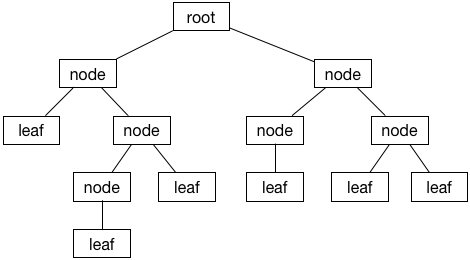## Terminology

Before we dive into the code, it’s important that you understand some important terminology first.

On top of all the terms related to a general purpose tree, a binary tree adds the notion of left and right children.

### Left Child

The left child descends from the left side: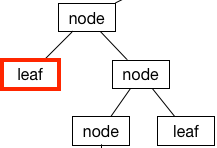### Right Child

Surprisingly, the right side is the right child: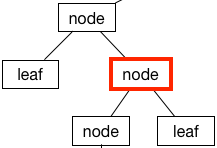### Leaf Node

If a node doesn’t have any children, it’s called a leaf node: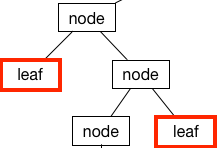### Root

The root is the node at the top of the tree (programmers like their trees upside down):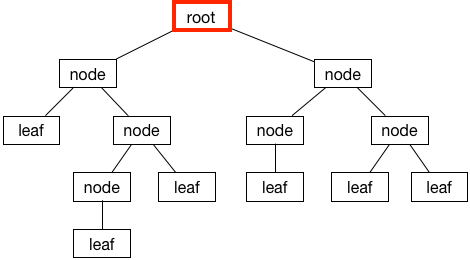## Binary Tree Implementation in Swift

Like other trees, a binary tree is composed of nodes. One way to represent a node is using a class (don’t enter this into a Playground yet, this is just an example):

```class Node<T> {
var value: T
var leftChild: Node?
var rightChild: Node?

init(value: T) {
self.value = value
}
}
```

In a binary tree, every node holds some data (`value`), and has a left and right child (`leftChild` and `rightChild`). In this implementation, `leftChild` and `rightChild` are optionals, meaning they can be `nil`.

That’s the traditional way to build trees. However, the thrill seeker you are shall rejoice today, because you’ll try something new! :]

### Value Semantics

One of the core ideas of Swift is using value types (like `struct` and `enum`) instead of reference types (like `class`) where appropriate. Well, creating a binary tree is a perfect case to use a value type – so in this tutorial, you’ll you’ll implement the binary tree as an enum type.

Note: To learn more about reference types vs. value types in Swift, check out our Reference vs. Value Types in Swift series.

Create a new Swift playground (this tutorial uses Xcode 8 beta 5) and add the following enum declaration:

```enum BinaryTree<T> {

}
```

You’ve declared a enum named `BinaryTree`. The syntax declares this to be a generic enum that allows it to infer it’s own type information at the call site.

### States

Enumerations are rigid, in that they can only be in one state or another. Fortunately, this fits into the idea of binary trees quite elegantly. A binary tree is a finite set of nodes that is either empty, or consists of the value at the node and references to it’s left and right children.

```enum BinaryTree<T> {
case empty
case node(BinaryTree, T, BinaryTree)
}
```

If you’re coming from another programming language, the `node` case may seem a bit foreign. Swift enums allow for associated values, which is a fancy term for saying you can attach stored properties with a case.

In `node(BinaryTree, T, BinaryTree)`, the parameter types inside the brackets correspond to the left child, value, and the right child, respectively.

That’s a fairly compact way of modelling a binary tree. However, you’re immediately greeted with a compiler error:

```Recursive enum 'BinaryTree<T>' is not marked 'indirect'
```

Xcode should make an offer to fix this for you. Accept it, and your enum should now look like this:

```indirect enum BinaryTree<T> {
case empty
case node(BinaryTree, T, BinaryTree)
}
```

### Indirection

Enumerations in Swift are value types. When Swift tries to allocate memory for value types, it needs to know exactly how much memory it needs to allocate.

The enumeration you’ve defined is a recursive enum. That’s an enum that has an associated value that refers to itself. Recursive value types have a indeterminable size.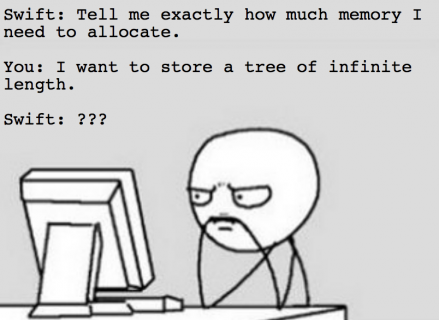So you’ve got a problem here. Swift expects to know exactly how big the enum is, but the recursive enum you’ve created doesn’t expose that information.

Here’s where the `indirect` keyword comes in. `indirect` applies a layer of indirection between two value types. This introduces a thin layer of reference semantics to the value type.

The enum now holds references to it’s associated values, rather than their value. References have a constant size, so you no longer have the previous problem.

While the code now compiles, you can be a little bit more concise. Update `BinaryTree` to the following:

```enum BinaryTree<T> {
case empty
indirect case node(BinaryTree, T, BinaryTree)
}
```

Since only the `node` case is recursive, you only need to apply `indirect` to that case.

## Example: Sequence of Arithmetical Operations

An interesting exercise to check out is to model a series of calculations using a binary tree. Take this for an example for modelling `(5 * (a - 10)) + (-4 * (3 / b))`: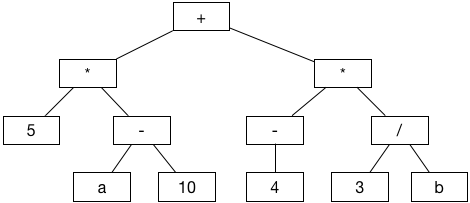Write the following at the end of your playground file:

```// leaf nodes
let node5 = BinaryTree.node(.empty, "5", .empty)
let nodeA = BinaryTree.node(.empty, "a", .empty)
let node10 = BinaryTree.node(.empty, "10", .empty)
let node4 = BinaryTree.node(.empty, "4", .empty)
let node3 = BinaryTree.node(.empty, "3", .empty)
let nodeB = BinaryTree.node(.empty, "b", .empty)

// intermediate nodes on the left
let Aminus10 = BinaryTree.node(nodeA, "-", node10)
let timesLeft = BinaryTree.node(node5, "*", Aminus10)

// intermediate nodes on the right
let minus4 = BinaryTree.node(.empty, "-", node4)
let divide3andB = BinaryTree.node(node3, "/", nodeB)
let timesRight = BinaryTree.node(minus4, "*", divide3andB)

// root node
let tree = BinaryTree.node(timesLeft, "+", timesRight)
```

You need to build up the tree in reverse, starting with the leaf nodes and working your way up to the top.

### CustomStringConvertible

Verifying a tree structure can be hard without any console logging. Swift has a handy protocol named `CustomStringConvertible`, which allows you define a custom output for `print` statements. Add the following code just below your `BinaryTree` enum:

```extension BinaryTree: CustomStringConvertible {
var description: String {
switch self {
case let .node(left, value, right):
return "value: \(value), left = [" + left.description + "], right = [" + right.description + "]"
case .empty:
return ""
}
}
}
```

Print the tree by writing the following at the end of the file:

```print(tree)
```

You should see something like this:

```value: +, left = [value: *, left = [value: 5, left = [], right = []], right = [value: -, left = [value: a, left = [], right = []], right = [value: 10, left = [], right = []]]], right = [value: *, left = [value: -, left = [], right = [value: 4, left = [], right = []]], right = [value: /, left = [value: 3, left = [], right = []], right = [value: b, left = [], right = []]]]
```

With a bit of imagination, you can see the tree structure. ;-) It helps if you indent it:

```value: +,
left = [value: *,
left = [value: 5, left = [], right = []],
right = [value: -,
left = [value: a, left = [], right = []],
right = [value: 10, left = [], right = []]]],
right = [value: *,
left = [value: -,
left = [],
right = [value: 4, left = [], right = []]],
right = [value: /,
left = [value: 3, left = [], right = []],
right = [value: b, left = [], right = []]]]
```

### Getting The Count

Another useful feature is being able to get the number of nodes in the tree. Add the following just inside your `BinaryTree` enumeration:

```var count: Int {
switch self {
case let .node(left, _, right):
return left.count + 1 + right.count
case .empty:
return 0
}
}
```

Test it out by adding this to the end of your playground:

```tree.count
```

You should see the number 12 in the sidebar, since there are 12 nodes in the tree.

Great job making it this far. Now that you’ve got a good foundation for binary trees, it’s time to get acquainted with the most popular tree by far – the Binary Search Tree!

## Binary Search Trees

A binary search tree is a special kind of binary tree (a tree in which each node has at most two children) that performs insertions and deletions such that the tree is always sorted.

### “Always Sorted” Property

Here is an example of a valid binary search tree: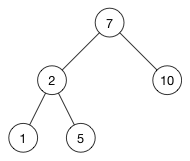Notice how each left child is smaller than its parent node, and each right child is greater than its parent node. This is the key feature of a binary search tree.

For example, 2 is smaller than 7 so it goes on the left; 5 is greater than 2 so it goes on the right.

### Insertion

When performing an insertion, starting with the root node as the current node:

• If the current node is empty, you insert the new node here.
• If the new value is smaller, you go down the left branch.
• If the new value is greater, you go down the right branch.

You traverse your way down the tree until you find an empty spot where you can insert the new value.

For example, imagine you want to insert the value 9 into the above tree:

1. Start at the root of the tree (the node with the value 7), and compare it to the new value 9.
2. 9 > 7, so you go down the right branch
3. Compare 9 with 10. Since 9 < 10, go down the left branch.
4. This left branch is empty, thus you’ll insert a new node for 9 at this location.

The new tree now looks like this: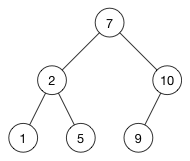Here’s another example. Imagine you want to insert 3 into the above tree:

1. Start at the root of the tree (the node with the value 7), and compare it to the new value 3.
2. 3 < 7, so you go down the left branch
3. Compare 3 with 2. Since 3 > 2, go down the right branch.
4. Compare 3 with 5. Since 3 < 5, go down the left branch.
5. The left branch is empty, thus you’ll insert a new node for 3 at this location.

The new tree now looks like this: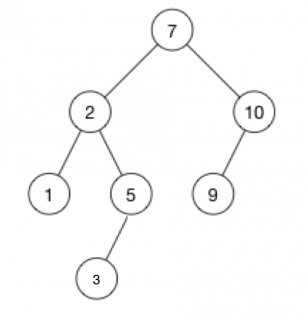There is always only one possible place where the new element can be inserted in the tree. Finding this place is usually pretty quick. It takes O(h) time, where h is the height of the tree.

Note: If you’re not familiar with the height of a tree, check out the previous article on Swift Trees.

### Challenge: Implementing Insertion

Now that you’ve got an idea of how insertion works, it’s implementation time. Add the following method to your `BinaryTree` enum:

```// 1.
mutating func naiveInsert(newValue: T) {
// 2.
guard case .node(var left, let value, var right) = self else {
// 3.
self = .node(.empty, newValue, .empty)
return
}

// 4. TODO: Implement rest of algorithm!

}
```

Let’s go over this section by section:

1. Value types are immutable by default. If you create a method that tries to mutate something within the value type, you’ll need to explicitly specify that by prepending the `mutating` keyword in front of your method.
2. You’re using the `guard` statement to expose the left child, current value, and right child of the current node. If this node is `empty`, then `guard` will fail into it’s `else` block.
3. In this block, `self` is `empty`. You’ll insert the new value here.
4. This is where you come in – hang tight for a second.

In a moment, you will try to implement section 4 based on the algorithm discussed above. This is a great exercise not only for understanding binary search trees, but also honing your recursion skills.

But before you do, you need to make a change to the `BinaryTree` signature. In section 4, you’ll need to compare whether the new value with the old value, but you can’t do this with the current implementation of the binary tree. To fix this, update the `BinaryTree` enum to the following:

```enum BinaryTree<T: Comparable> {
// stuff inside unchanged
}
```

The `Comparable` protocol enforces a guarantee that the type you’re using to build the binary tree can be compared using the comparison operators, such as the `<` operator.

Now, go ahead and try to implement section #4 based on the algorithm above. Here it is again for your reference:

• If the current node is empty, you insert the new node here. Done!
• If the new value is smaller, you go down the left branch. You need to do this.
• If the new value is greater, you go down the right branch. You need to do this.

If you get stuck, you can check the solution below.

[spoiler title="Solution"]

```// 4. TODO: Implement naive algorithm!
if newValue < value {
left.naiveInsert(newValue: newValue)
} else {
right.naiveInsert(newValue: newValue)
}
```

[/spoiler]

### Copy on Write

Though this is a great implementation, it won't work. Test this by writing the following at the end of your playground:

```var binaryTree: BinaryTree<Int> = .empty
binaryTree.naiveInsert(newValue: 5) // binaryTree now has a node value with 5
binaryTree.naiveInsert(newValue: 7) // binaryTree is unchanged
binaryTree.naiveInsert(newValue: 9) // binaryTree is unchanged
```Copy-on-write is the culprit here. Every time you try to mutate the tree, a new copy of the child is created. This new copy is not linked with your old copy, so your initial binary tree will never be updated with the new value.

This calls for a different way to do things. Write the following at the end of the `BinaryTree` enum:

```private func newTreeWithInsertedValue(newValue: T) -> BinaryTree {
switch self {
// 1
case .empty:
return .node(.empty, newValue, .empty)
// 2
case let .node(left, value, right):
if newValue < value {
return .node(left.newTreeWithInsertedValue(newValue: newValue), value, right)
} else {
return .node(left, value, right.newTreeWithInsertedValue(newValue: newValue))
}
}
}
```

This is a method that returns a new tree with the inserted element. The code is relatively straightforward:

1. If the tree is empty, you want to insert the new value here.
2. If the tree isn't empty, you'll need to decide whether to insert into the left or right child.

Write the following method inside your `BinaryTree` enum:

```mutating func insert(newValue: T) {
self = newTreeWithInsertedValue(newValue: newValue)
}
```

Test your code by replacing the test lines at the bottom of your playground:

```binaryTree.insert(newValue: 5)
binaryTree.insert(newValue: 7)
binaryTree.insert(newValue: 9)
```

You should end up with the following tree structure:

```value: 5,
left = [],
right = [value: 7,
left = [],
right = [value: 9,
left = [],
right = []]]
```

Congratulations - now you've got insertion working!

### Insertion Time Complexity

As discussed in the spoiler section, you need to create a new copy of the tree every time you make an insertion. Creating a new copy requires going through all the nodes of the previous tree. This gives the insertion method a time complexity of O(n).

Note: Average time complexity for a binary search tree for the traditional implementation using classes is O(log n), which is considerably faster. Using classes (reference semantics) won't have the copy-on-write behaviour, so you'll be able to insert without making a complete copy of the tree.

## Traversal Algorithms

Traversal algorithms are fundamental to tree related operations. A traversal algorithm goes through all the nodes in a tree. There are three main ways to traverse a binary tree:

### In-order Traversal

In-order traversal of a binary search tree is to go through the nodes in ascending order. Here's what it looks like to perform an in-order traversal: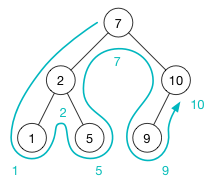Starting from the top, you head to the left as much as you can. If you can't go left anymore, you'll visit the current node and attempt to traverse to the right side. This procedure continues until you traverse through all the nodes.

Write the following inside your `BinaryTree` enum:

```func traverseInOrder(process: @noescape (T) -> ()) {
switch self {
// 1
case .empty:
return
// 2
case let .node(left, value, right):
left.traverseInOrder(process: process)
process(value)
right.traverseInOrder(process: process)
}
}
```

This code is fairly straightforward:

1. If the current node is empty, there's no way to go down further. You'll simply return here.
2. If the current node is non empty, then you can go down further. The definition of in-order traversal is to go down the left side, visit the node, and then the right side.

To see this in action, you'll create the binary tree shown above. Delete all the test code at the bottom of your playground and replace it with the following:

```var tree: BinaryTree<Int> = .empty
tree.insert(newValue: 7)
tree.insert(newValue: 10)
tree.insert(newValue: 2)
tree.insert(newValue: 1)
tree.insert(newValue: 5)
tree.insert(newValue: 9)

tree.traverseInOrder { print(\$0) }
```

You've created a binary search tree using your insert method. `traverseInOrder` will go through your nodes in ascending order, passing the value in each node to the trailing closure.

Inside the trailing closure, you're printing the value that was passed in by the traversal method. `\$0` is a shorthand syntax that references the parameter that is passed in to the closure.

You should see the following output in your console:

```1
2
5
7
9
10
```

### Pre-order Traversal

Pre-order traversal of a binary search tree is to go through the nodes whilst visiting the current node first. The key here is calling `process` before traversing through the children. Write the following inside your `BinaryTree` enum:

```func traversePreOrder( process: @noescape (T) -> ()) {
switch self {
case .empty:
return
case let .node(left, value, right):
process(value)
left.traversePreOrder(process: process)
right.traversePreOrder(process: process)
}
}
```

### Post-order Traversal

Post-order traversal of a binary search tree is to visit the nodes only after traversing through it's left and right children. Write the following inside your `BinaryTree` enum:

```func traversePostOrder( process: @noescape (T) -> ()) {
switch self {
case .empty:
return
case let .node(left, value, right):
left.traversePostOrder(process: process)
right.traversePostOrder(process: process)
process(value)
}
}
```

These 3 traversal algorithms serve as a basis for many complex programming problems. Understanding them will prove useful for many situations, including your next programming interview!

### Mini Challenge

What is the time complexity of the traversal algorithms?

[spoiler title="Solution"]
The time complexity is O(n), where n is the number of nodes in the tree.

This should be obvious, since the idea of traversing a tree is to go through all the nodes!
[/spoiler]

## Searching

As the name suggests, a binary search tree is known best for facilitating efficient searching. A proper binary search tree will have all it's left children less than it's parent node, and all it's right children equal to or greater than it's parent node.

By exploiting this guarantee, you'll be able to determine which route to take - the left child, or the right child - to see if your value exists within the tree. Write the following inside your `BinaryTree` enum:

```func search(searchValue: T) -> BinaryTree? {
switch self {
case .empty:
return nil
case let .node(left, value, right):
// 1
if searchValue == value {
return self
}

// 2
if searchValue < value {
return left.search(searchValue: searchValue)
} else {
return right.search(searchValue: searchValue)
}
}
}
```

Much like the traversal algorithms, searching involves traversing down the binary tree:

1. If the current value matches the value you're searching for, you're done searching. Return the current subtree
2. If execution continues to this point, it means you haven't found the value. You'll need to decide whether you want to go down to the left or right. You'll decide using the rules of the binary search tree.

Unlike the traversal algorithms, the search algorithm will traverse only 1 side at every recursive step. On average, this leads to a time complexity of O(log n), which is considerably faster than the O(n) traversal.

You can test this by adding the following to the end of your playground:

```tree.search(searchValue: 5)
```

## Where To Go From Here?

I hope you enjoyed this tutorial on making a Swift Binary Tree data structure!

Here is a Swift playground with the above code. You can also find alternative implementations and further discussion in the Binary Search Tree section of the Swift Algorithm Club repository.

This was just one of the many algorithms in the Swift Algorithm Club repository. If you're interested in more, check out the repo.

It's in your best interest to know about algorithms and data structures - they're solutions to many real world problems, and are frequently asked as interview questions. Plus it's fun!

So stay tuned for many more tutorials from the Swift Algorithm club in the future. In the meantime, if you have any questions on implementing trees in Swift, please join the forum discussion below!

Note: The Swift Algorithm Club is always looking for more contributors. If you've got an interesting data structure, algorithm, or even an interview question to share, don't hesitate to contribute! To learn more about the contribution process, check out our Join the Swift Algorithm Club article.If you enjoyed what you learned in this tutorial, why not check out our Data Structures and Algorithms in Swift book, available on our store?

In Data Structures and Algorithms in Swift, you’ll learn how to implement the most popular and useful data structures and when and why you should use one particular datastructure or algorithm over another. This set of basic data structures and algorithms will serve as an excellent foundation for building more complex and special-purpose constructs.

As well, the high-level expressiveness of Swift makes it an ideal choice for learning these core concepts without sacrificing performance.

• You’ll start with the fundamental structures of linked lists, queues and stacks, and see how to implement them in a highly Swift-like way.
• Move on to working with various types of trees, including general purpose trees, binary trees, AVL trees, binary search trees and tries.
• Go beyond bubble and insertion sort with better-performing algorithms, including mergesort, radix sort, heap sort and quicksort.
• Learn how to construct directed, non-directed and weighted graphs to represent many real-world models, and traverse graphs and trees efficiently with breadth-first, depth-first, Dijkstra’s and Prim’s algorithms to solve problems such as finding the shortest path or lowest cost in a network.
• And much, much more!

By the end of this book, you’ll have hands-on experience solving common issues with data structures and algorithms — and you’ll be well on your way to developing your own efficient and useful implementations.